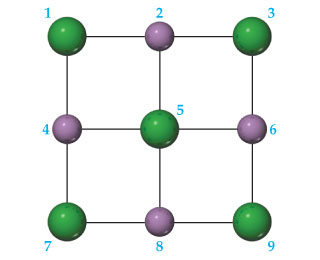# Problem: A portion of a two-dimensional "slab" of NaCl(s) is shown here in which the ions are numbered.If this pattern of ions was extended indefinitely in two dimensions, would the lattice energy be positive or negative?

###### FREE Expert Solution

We have to determine if the given “slab”, when extended indefinitely in two dimensions, would result into a positive or negative lattice energy.

Lattice energy is the energy released when gaseous ions are brought closer to form a crystalline solid.###### Problem Details
A portion of a two-dimensional "slab" of NaCl(s) is shown here in which the ions are numbered.If this pattern of ions was extended indefinitely in two dimensions, would the lattice energy be positive or negative?